# Number Systems

## Number Systems with Examples:

In this article, we will learn about Number Systems with Examples. Computers work upon binary number systems but we understand decimal number systems. So, it is difficult for us to adjust to the binary number system. So, other number systems are also there.

##### What is the importance of the Number System?

If you are doing some paperwork and if you are involved in some complex logic then you may need to know the number system to some extent. And in academics, this is taught. Basically, for learning C/C++ programming, the idea of the number system is sufficient. So let us start learning about number systems. We have 4 types of number systems: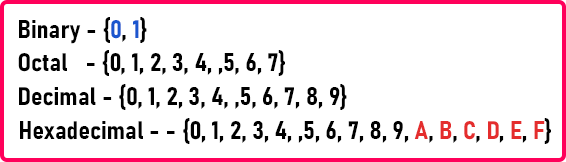In our daily life, we use the decimal number system. In the decimal number system, the figures are from 0 to 9. We are calling them figures. The binary number system has only two figures that are 0 and 1. As we said it is difficult to work with a binary number system so two more number systems are introduced. One is the octal number system which has figures from 0 to 7. And another is the hexadecimal number system that contains figures from 0 to 9 plus A to F alphabets. So, in total it has 16 figures.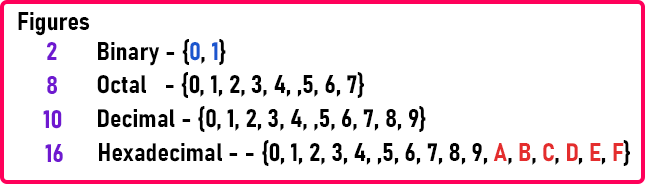We all are familiar with binary and decimal number systems. Octal and hexadecimal number systems are useful for understanding or working with binary. For understanding these comparatively, we have created a table,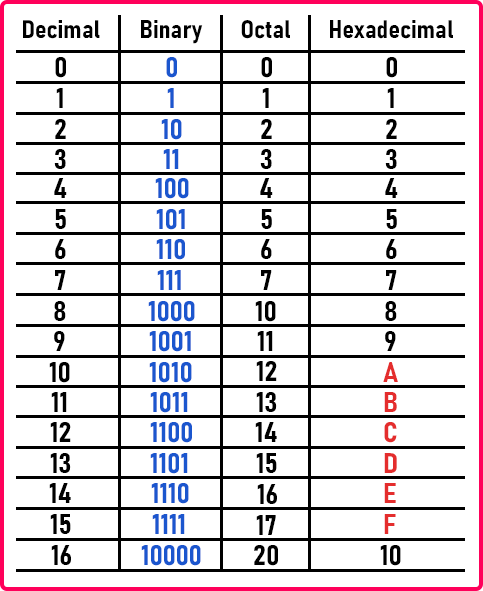##### Decimal Number System:

In this table, the 1st column is of decimal numbers that are written from 0 to 16. See 16 is value means figures are 1 and 6. For 15, figures are 1 and 5. So if you observe decimal numbers here, we have digits that are figures from 0 to 9 and once we run out of digits, we start writing a figure or a digit in 2 digits or 2 figures. So, after 9 is 10 that is 1 and 0. On the left-hand side, we have 1 but on the right-hand side, that is the unit side we again freshly started from 0 onwards. So, it is like carrying. So, if we add 9 and 1 it will be 10.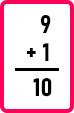The last figure that we have is 9. We don’t have the figure for 10. So, we should write 0 and take carry 1. Then we get 10 on addition. Here figures are 1, 0 and the value is 10 in the decimal number system.

##### Octal Number System:

Now, similarly, let us look at the octal number system. In octal, the last figure that we have is 7. Value and figure both are 7. So, the next should be 8. If we add 1 to the 7 then it should be 8. But we don’t have 8 in the octal number system. So, we have to move on to the 2-digit number.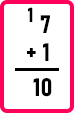So here we have written 0 is a fresh start and take carry 1. Then it will be 10. Here figures are 1, 0 but the value is 8 in the octal number system. To differentiate between decimal and octal number systems we can mention the base as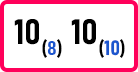Here 10 with base 8 is in the octal number system and 10 with base 10 is in the decimal number system.

Now let us understand the hexadecimal number system. In this system, we have figures from 0 to 9. For value 10, we write A, for value 11, we write B, for value 12, we write C, and so on till F. for value 15, we write F. So, F is the last figure in the hexadecimal number system. So, if we add 1 to F then, the value of the result will be 16. Because figure F means the value is 15 so if we add 1 to 15 then the value will be 16. So let us see how to write 16 in hexadecimal.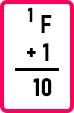There is no figure for 16 so we have written 0 which is a fresh start and taking 1 as carrying. So, it will become 10. See here the figure is 1, 0 but the value is 16.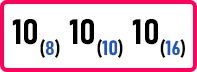So, we can write 16 as 10 with base 16 in the hexadecimal number system. With the base, we can differentiate between the number systems. Now let us come to the binary number system.

##### Binary Number System:

Let us add 1 to 0,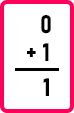It gives result 1. Now if we add 1 to 1 then we will move to the value 2. We know 1 + 1 will gives the value 2. But we don’t have the figure for 2 in the binary number system. We have figures 0 and 1 only.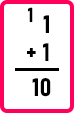Here again, we did the same thing. We write the 0 that is a fresh start and take 1 as carrying. So, it will give 10. Here, the figure is 1, 0 but the value is 2.

We can differentiate between these figures by writing the base. So, figure 1, 0 in decimal means the value is also 10, figure 1, 0 in octal means the value is 8, figure 1, 0 in hexadecimal means the value is 16, and figure 1, 0 in binary number system means the value is 2. So, in the above table, you can compare the values in the different number systems. We know the figure for value 2 is 10 in binary. So, if we add 2 with 1,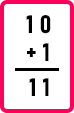We know 0 + 1 is 1, so we write 1 and the other 1 remains the same as there is no carry. So, here we get 11. This means the figure is 1, 1 but the value is 3. Now if we add 1 with 3,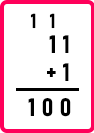First, we add 1 + 1. We know 1 + 1 is 10 in binary as we did it above. So, we write 0 in the result and take 1 carry. Again 1 + 1 is 10 so we write 0 in the result and take 1 carry. As there is no more figure just write down the carry in the result. So, we get 100. Figure 1, 0, 0 means the value is 4. Now let us see the basic operations. In binary,

0 + 0 = 0

0 + 1 = 1

1 + 0 = 1

1 + 1 = 10

These are basic operations in a binary number system. Similarly, by adding 1 to a binary number, we will get the next binary number. Now let us take one more example in binary. We want to add 1 with a value of 11. So, 11 is written as 1011 in binary. So let us perform the addition.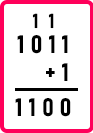Here we have performed addition between 1 and 1011 in the binary system. First, we perform 1 + 1. We already know the result which is 10. So, we write 0 in the result and take 1 as carry. Then again 1 + 1 will give 10. So again, we write 0 in the result and 1 as carry. Now 1 + 0 that is 1. So, we write 1 in the result. And the last 1 will remain the same in the result as there is no carry. So, the final result is 1100. Figure 1, 1, 0, 0 means the value is 12.

You should be able to tell the binary form from 1 to 16 values. This will help you in other subjects of computer science. Hexadecimal is also important but octal is not much used. Now we will give you the basic idea of conversion then in upcoming articles we will explain to you the conversion between one number system into another number system.

Let us take a decimal number, suppose we have (349)10. We can write 349 as 300 + 40 + 9

Also, 3 x 100 + 4 x 10 + 9 x 1

Then, 3 x 102 + 4 x 101 + 9 x 100

As we go on increasing the digits, it will be raising to powers of 10. If we multiply these and add them then we will get the same number which is 349. So, if we go reverse, we will get the same number. Now suppose we have a binary number that is (101011101)2. We can write it as,

1 x 28 + 0 x 27 + 1 x 26 + 0 x 25 + 1 x 24 + 1 x 23 + 1 x 22 + 0 x 21 + 1 x 20

So, if we solve the above equation then we will get the decimal number. We can write it as,

256 + 0 + 64 + 0 + 16 + 8 + 4 + 0 + 1 = 349

So just here we want to give the basic idea that if a number is in the decimal system, then it is in powers of 10 and if a number is in the binary system, then it is in powers of 2. So, this is the basic idea behind the conversion of the number system.